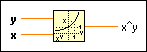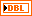# Power Of X Function

## LabVIEW 2018 Help

Edition Date: March 2018
Part Number: 371361R-01
View Product InfoLabVIEW 2015 HelpLabVIEW 2016 HelpLabVIEW 2017 HelpLabVIEW 2018 HelpLabVIEW 2019 Help

Owning Palette: Exponential Functions

Requires: Base Development System

Computes x raised to the y power.

If x is not complex, it must be greater than zero unless y is an integer value. Otherwise, the result is NaN. If y is 0, x^y is 1 for all values of x, including 0. The connector pane displays the default data types for this polymorphic function.y can be a scalar number, array or cluster of numbers, array of clusters of numbers, and so on.x can be a scalar number, array or cluster of numbers, array of clusters of numbers, and so on.x^y is x to the power of y. When x and y are complex, the following equation defines x^y: x^y = exp(y * ln(x)) Refer to the Exponential function and the Natural Logarithm function for more information.

## Power Of X Details

When you wire matrix data as an input to this function, a VI that includes subVIs that work with the matrix data type replaces the function. The resulting VI has the same icon but contains a matrix-specific algorithm. The node remains a VI if you disconnect the matrix from the input(s). Wire other data types as inputs to restore the original function. If you wire a data type to a function and that data type causes a basic math operation to fail, the function returns an empty matrix or NaN.